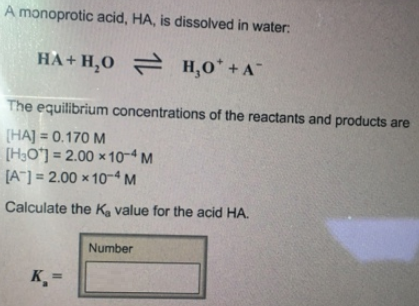# Problem: A monoprotic acid, HA, is dissolved in water: HA + H2O ⇌ H3O+ + A-The equilibrium concentrations of the reactants and products are [HA] = 0.170 M [H3O+] = 2.00 × 10-4 M [A] = 2.00 × 10-4 M Calculate the Ka value for the acid HA.

###### FREE Expert Solution
94% (222 ratings)###### Problem Details

A monoprotic acid, HA, is dissolved in water:

HA + H2O ⇌ H3O+ + A-

The equilibrium concentrations of the reactants and products are

[HA] = 0.170 M

[H3O+] = 2.00 × 10-4

[A] = 2.00 × 10-4

Calculate the Ka value for the acid HA.